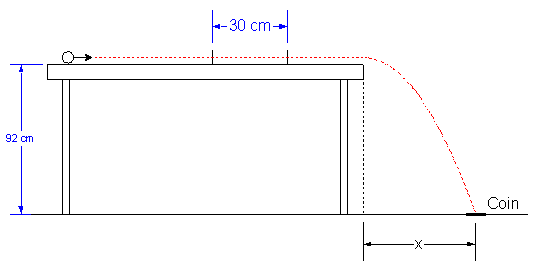# Marble Rolling/Projctle Motion

1. A student finds that it takes .20s for a ball to pass through photogates places 30cm apart on a level ramp. The end of the ramp is 92cm above the floor. Where should a coin be placed so that the ball strikes it directly on impact with the ground?

2. ΔX=ViTf+1/2 ATf^2
v= ΔX/ΔT

3. Tf=.2s
h=92cm (.92m)
I don't understand why the 30cm is there or how to complete the problem.

## Answers and Replies

1. A student finds that it takes .20s for a ball to pass through photogates places 30cm apart on a level ramp. The end of the ramp is 92cm above the floor. Where should a coin be placed so that the ball strikes it directly on impact with the ground?

2. ΔX=ViTf+1/2 ATf^2
v= ΔX/ΔT

3. Tf=.2s
h=92cm (.92m)
I don't understand why the 30cm is there or how to complete the problem.

I cant understand the question but you might use 30sm to find radius ...

I cant understand the question but you might use 30sm to find radius ...

It's a projectile motion problem...you don't use radius ever.

gneill
Mentor#### AttachmentsYep, that's the picture I have! However, I have NO CLUE where to start it. Where does the 30cm come into play? I'm thinking find the time, then average velocity, to then both put into the ΔX=blah blah blah equation

gneill
Mentor
Yep, that's the picture I have! However, I have NO CLUE where to start it. Where does the 30cm come into play? I'm thinking find the time, then average velocity, to then both put into the ΔX=blah blah blah equation

You're GIVEN the time for it to pass between the photogates. No need to find it. If you know the time for the projectile to travel the 30 cm between the gates, what's its speed?

If you then have its speed, what are the formulas that describe its trajectory when it leaves the edge of the level surface?

You're GIVEN the time for it to pass between the photogates. No need to find it. If you know the time for the projectile to travel the 30 cm between the gates, what's its speed?

If you then have its speed, what are the formulas that describe its trajectory when it leaves the edge of the level surface?

This is how I did the problem-
H=1/2gt^2
.92m=1/2(9.8)t^2
T=.43s

V=ΔX/ΔT
30cm/.2s
V=1.5m

Then I plugged in both answers into:
ΔX=ViTf+1/2ATf^2
ΔX=1.5(.43)+1/2(0)tf^2
ΔX=.65m

Does this look right?

It's a projectile motion problem...you don't use radius ever.

woops ... I didnt read whole topic, I read rolling and thought and ...

This is how I did the problem-
H=1/2gt^2
.92m=1/2(9.8)t^2
T=.43s

V=ΔX/ΔT
30cm/.2s
V=1.5m

Then I plugged in both answers into:
ΔX=ViTf+1/2ATf^2
ΔX=1.5(.43)+1/2(0)tf^2
ΔX=.65m

Does this look right?

Yep its right ... sorry for my above confusing post ... this problem was not in the language i am used to ...

No worries!

NascentOxygen
Staff Emeritus
Science Advisor
This is how I did the problem-
H=1/2gt^2
.92m=1/2(9.8)t^2
T=.43s

V=ΔX/ΔT
30cm/.2s
V=1.5m /sec

Then I plugged in both answers into:
ΔX=ViTf+1/2ATf^2
ΔX=1.5(.43)+1/2(0)tf^2
ΔX=.65m

Does this look right?
Your method looks right.

This is how I did the problem-
H=1/2gt^2
.92m=1/2(9.8)t^2
T=.43s

V=ΔX/ΔT
30cm/.2s
V=1.5m

Then I plugged in both answers into:
ΔX=ViTf+1/2ATf^2
ΔX=1.5(.43)+1/2(0)tf^2
ΔX=.65m

Does this look right?

Couple questions on the problem still-
1)First, why did I have the use the H=1/2gt^2 equation to get T=.43s? I think it has something to do with the horizontal and vertical relationship to each other? But if the problem gives me .2s already-what is that for?

2) Second, I used the average velocity equation, but how can the average velocity switch to being the Vi in the ΔX=ViTf+1/2ATf^2 equation? How does that work?

Couple questions on the problem still-
1)First, why did I have the use the H=1/2gt^2 equation to get T=.43s? I think it has something to do with the horizontal and vertical relationship to each other? But if the problem gives me .2s already-what is that for?

2) Second, I used the average velocity equation, but how can the average velocity switch to being the Vi in the ΔX=ViTf+1/2ATf^2 equation? How does that work?

1)

when marble falls from table, in Y direction there is no initial velocity (right?) ... so for eqn:
y = uyt + 0.5 g t2 .. uy turns out to be 0

NascentOxygen
Staff Emeritus
Science Advisor
Couple questions on the problem still-
1)First, why did I have the use the H=1/2gt^2 equation to get T=.43s? I think it has something to do with the horizontal and vertical relationship to each other? But if the problem gives me .2s already-what is that for?
The 0.2 sec is used to calculate the speed of the ball before it starts falling. The 0.43 sec is the time it spends in midair. While it's free-falling, it also continues to move horizontally away from the table.

2) Second, I used the average velocity equation, but how can the average velocity switch to being the Vi in the ΔX=ViTf+1/2ATf^2 equation? How does that work?
The initial speed of the ball was calculated on a level surface. What is the value of the horizontal component of gravity acting to speed up the ball over this path?